# In each reaction box, place the best reagent and conditions from the list below. There is more than one possible route...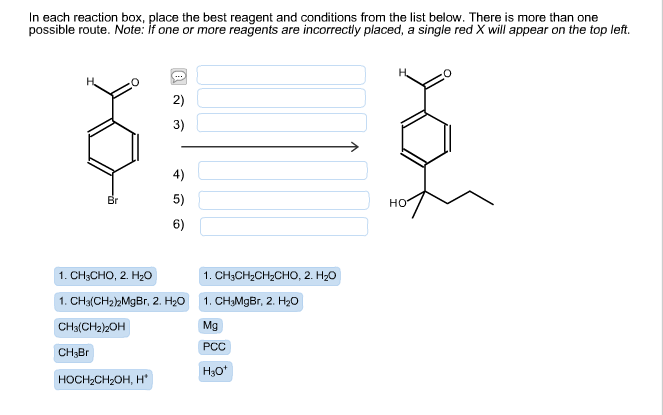In each reaction box, place the best reagent and conditions from the list below. There is more than one possible route. Note: If one or more reagents are incorrectly placed, a single red X will appear on the top left. CH3CHO, 2H2O CH2CH2CH2CHO, 2H2O CH3(CH2)2MgBr, H2O CH3MgBr, H2O CH3(CH2)2OH Mg CH3Br PCC HOCH2CH2OH, H+ H3O+

Concepts and reason

The concept used to solve this question is retrosynthetic analysis of the given product. In the retrosynthetic route, the last step of the synthesis is first determined and remaining steps can be fixed by functioning backwards from the product. Later, the given reagents can be placed in the order of conversion from starting material into product.

Fundamentals

Retrosynthetic analysis: In this analysis, the last step of the synthesis is first determined and remaining steps can be fixed by functioning backwards from the product. Simply telling, the synthesis of the target molecule in backward direction can be established in this strategy. This is also called as retrosynthesis (reverse synthesis). The retrosynthesis can be denoted by “ $\Rightarrow$ ” arrow.

Disconnection: It is an operation which breaks a bond in such a way that the target molecule converts into possible starting materials. Or it can be simply telling, it is reverse of chemical reaction.

FGI: In the retrosynthesis, functional group interconversion (FGI) takes place which means the conversion of one functional group into another functional group.

There are many numbers of routes to synthesize a target molecule but the most efficient way is taken into consideration.

The given staring material and the product (target molecule) is drawn as follows: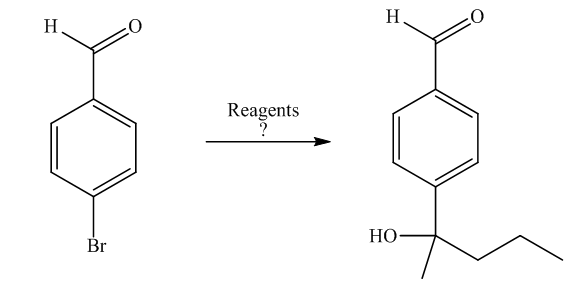The retrosynthesis of the target molecule is as follows:

The target molecule could be synthesized by the deprotection of acetal group of the tertiary alcohol. The tertiary alcohol could be synthesized by the treatment of ketone with methylmagnesium bromide.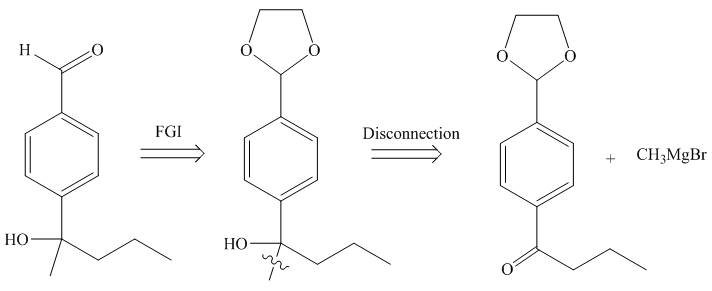The ketone could be synthesized by the oxidation of secondary alcohol which in turn could be synthesized by the treatment of aldehyde with arylmagnesium bromide.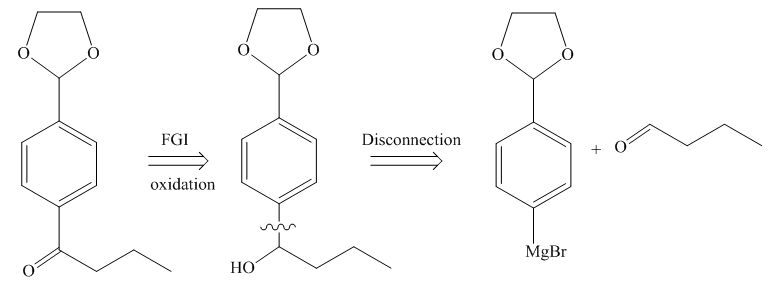The arylmagnesium bromide could be synthesized by the treatment of aromatic bromo compound with magnesium metal which in turn could be synthesized by the protection of 4-bromobenzaldehyde with ethylene glycol in the presence of acid.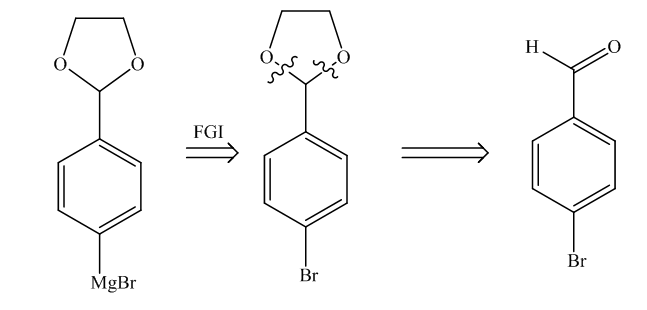From the retrosynthesis of target molecule in step 1, it is clear that the synthesis consists of 6 steps.

The reverse order of retrosynthesis represents the actual chemical synthesis.

Therefore, the synthesis of the target molecule can be written as follows: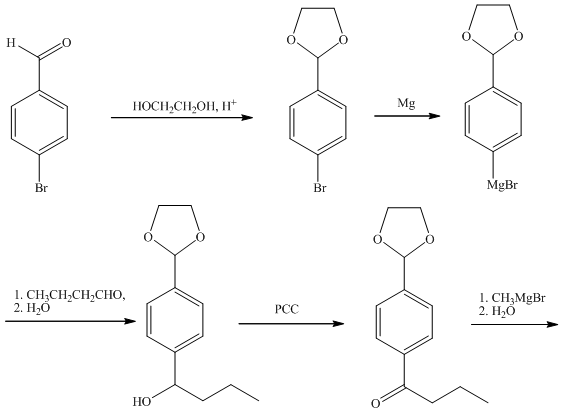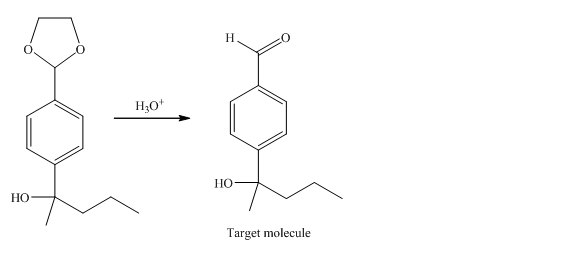And, hence the sequence of reagents required for the synthesis of target molecule from the starting material from the given list can be written as follows:

$\begin{array}{l}\\1){\rm{ OHC}}{{\rm{H}}_{\rm{2}}}{\rm{C}}{{\rm{H}}_{\rm{2}}}{\rm{OH, }}{{\rm{H}}^{\rm{ + }}}\\\\{\rm{2) Mg}}\\\\{\rm{3) 1}}{\rm{. C}}{{\rm{H}}_{\rm{3}}}{\rm{C}}{{\rm{H}}_{\rm{2}}}{\rm{C}}{{\rm{H}}_{\rm{2}}}{\rm{CHO, 2}}{\rm{. }}{{\rm{H}}_{\rm{2}}}{\rm{O}}\\\\{\rm{4) PCC}}\\\\{\rm{5) 1}}{\rm{. C}}{{\rm{H}}_{\rm{3}}}{\rm{MgBr, 2}}{\rm{. }}{{\rm{H}}_{\rm{2}}}{\rm{O}}\\\\{\rm{6) }}{{\rm{H}}_{\rm{3}}}{{\rm{O}}^{\rm{ + }}}\\\end{array}$

Ans:

The sequence of reagents required for the synthesis of target molecule from the starting material is as follows:

$\begin{array}{l}\\1){\rm{ OHC}}{{\rm{H}}_{\rm{2}}}{\rm{C}}{{\rm{H}}_{\rm{2}}}{\rm{OH, }}{{\rm{H}}^{\rm{ + }}}\\\\{\rm{2) Mg}}\\\\{\rm{3) 1}}{\rm{. C}}{{\rm{H}}_{\rm{3}}}{\rm{C}}{{\rm{H}}_{\rm{2}}}{\rm{C}}{{\rm{H}}_{\rm{2}}}{\rm{CHO, 2}}{\rm{. }}{{\rm{H}}_{\rm{2}}}{\rm{O}}\\\end{array}$

$\begin{array}{l}\\{\rm{4) PCC}}\\\\{\rm{5) 1}}{\rm{. C}}{{\rm{H}}_{\rm{3}}}{\rm{MgBr, 2}}{\rm{. }}{{\rm{H}}_{\rm{2}}}{\rm{O}}\\\\{\rm{6) }}{{\rm{H}}_{\rm{3}}}{{\rm{O}}^{\rm{ + }}}\\\end{array}$

##### Add Answer to: In each reaction box, place the best reagent and conditions from the list below. There is more than one possible route...
Similar Homework Help Questions
• ### In each reaction box, place the best reagent and conditions from the list below. In each reaction box. place the b...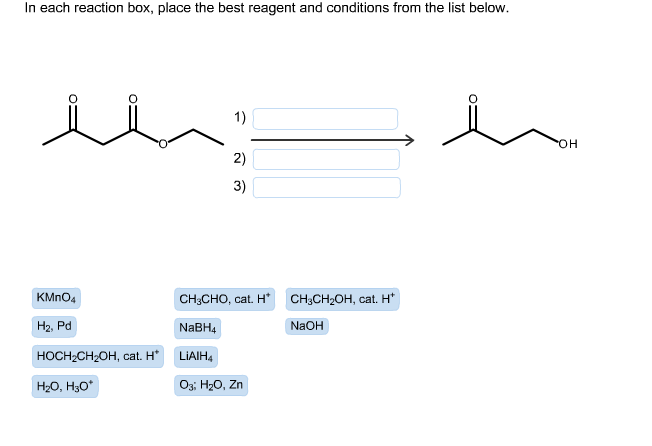In each reaction box, place the best reagent and conditions from the list below. In each reaction box. place the best reagent and conditions from the list below KMnO4 CH3CHO, cat. H+ CH3CH2OH, cat. H+ H2,Pd NaBH4 NaOH HOCH2CH2OH, cat. H+ LiAlH4 H2O, H3O+ O3: HO, Zn'

• ### in each reaction box place the best reagent and conditions from the list below In each...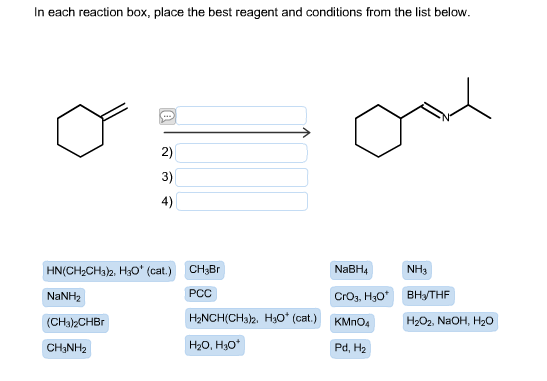in each reaction box place the best reagent and conditions from the list below In each reaction box, place the best reagent and conditions from the list below. NaBH4 HN(CH2CH312, H30 (cat.) CH3Br NH3 PCC NaNH2 CrO3 H3O BH3 THF H2NCH(CH312, H30 (cat.) KMno4 H202, NaOH, H20 (CH3)2CHBr H20, H30 CH3NH2 Pd, H2

• ### in each reaction box place the best reagent and conditions from the list below. lialh4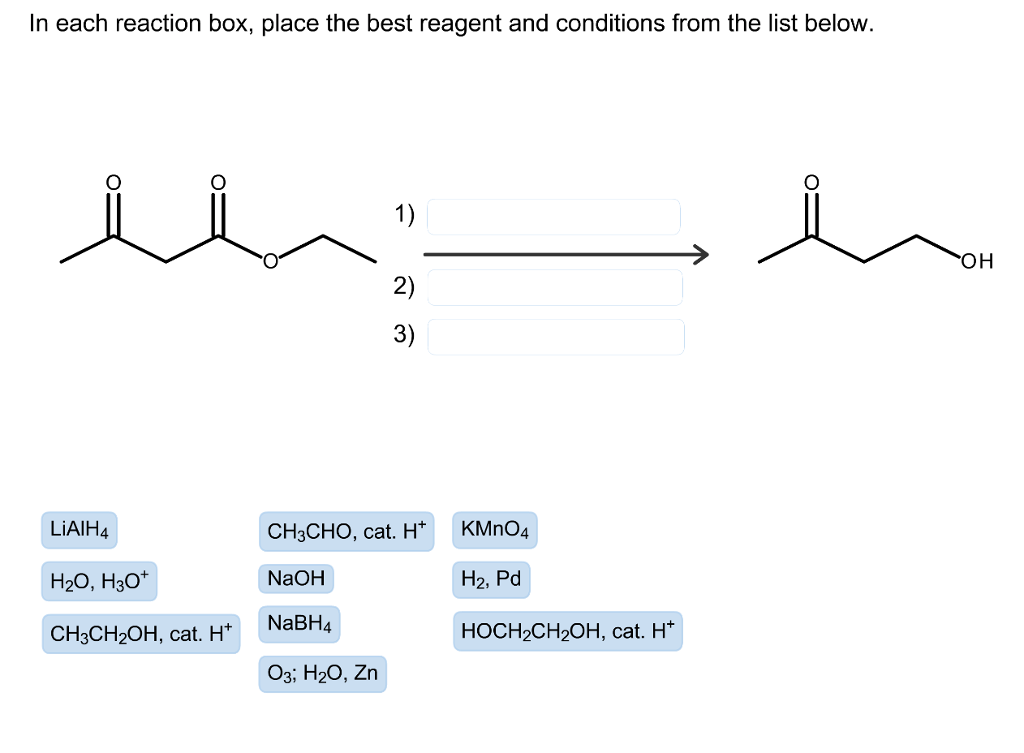In each reaction box, place the best reagent and conditions from the list below. 1) 2) 3) ОН LiAlH4 H20, H30 CH3CH20H, cat. H NaBH4 CH3CHO, cat. HKMnO4 NaOH H2, Pd HOCH2CH2OH, cat. H* Од; H2O, Zn Show transcribed image text

• ### In each reaction box, place the best reagent and conditions from the list below. In each reaction box, place the bes...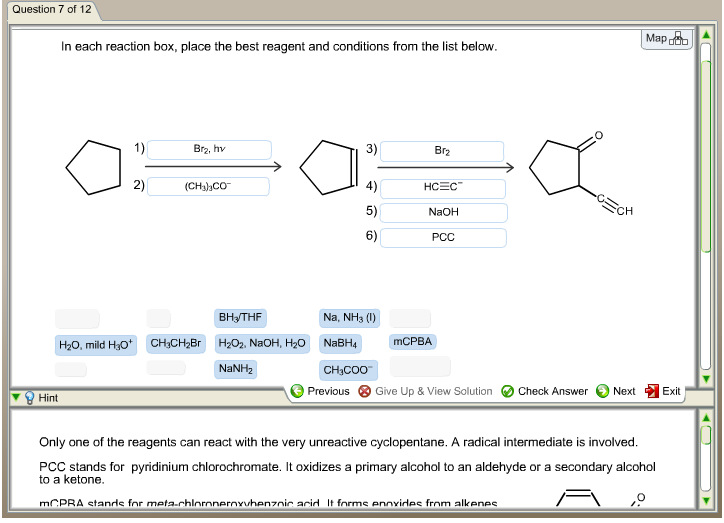In each reaction box, place the best reagent and conditions from the list below. In each reaction box, place the best reagent and conditions from the list below. Only one of the reagents can react with the very unreactive cyclopentane. A radical intermediate is involved. PCC stands for pyridinium chlorochromate. It oxidizes a primary alcohol to an aldehyde or a secondary alcohol to a ketone.

• ### In each reaction box, place the best reagent and conditions from the list below. In each...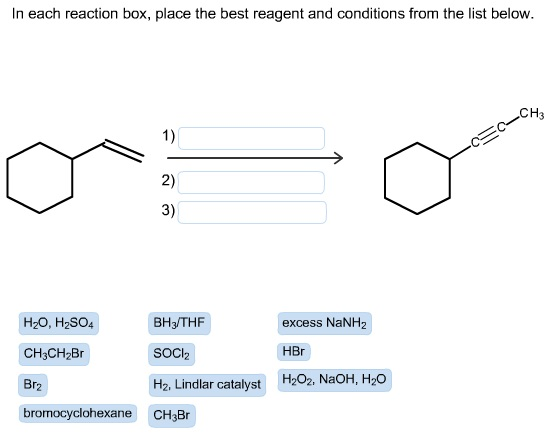In each reaction box, place the best reagent and conditions from the list below. In each reaction box, place the best reagent and conditions from the list below. CH3 BH3 THF H20, H2SO4 excess NaNH2 HBr SOCI2 CH3CH2Br H2, Lindlar catalyst H202, NaoH, H20 Br2 bromocyclohexane CH3Br

• ### In each reaction box, place the best reagent and conditions from the list below. 036 H2O...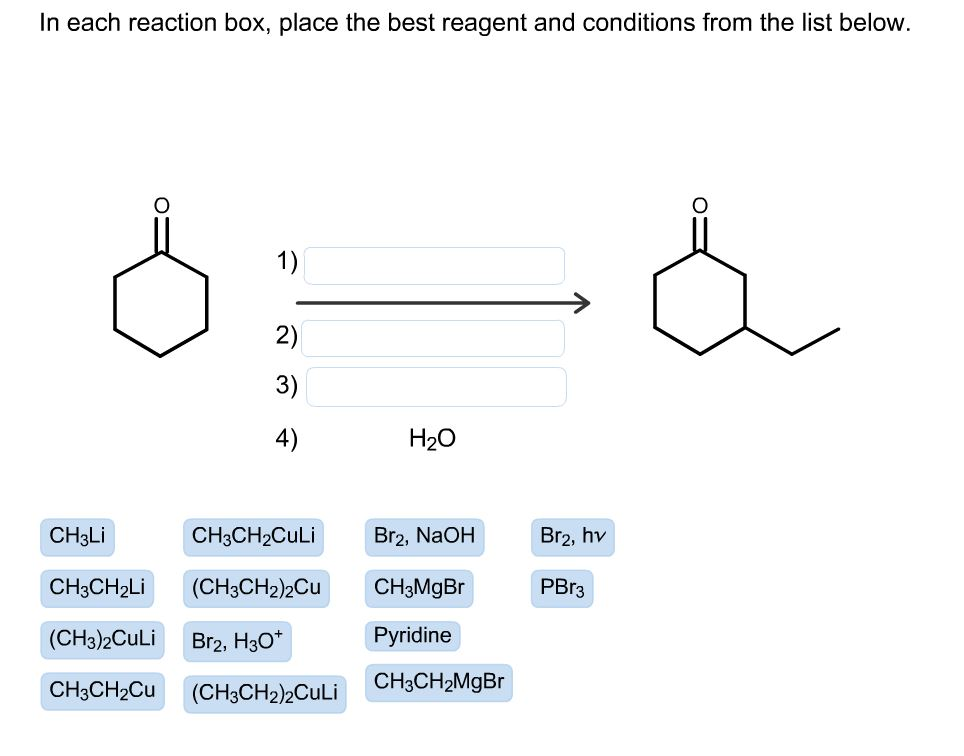In each reaction box, place the best reagent and conditions from the list below. 036 H2O CH3Li CH3CH2Culi Br2, NaOH Br2, hv CH3CH2Li (CH3CH2)2Cu CH3MgBr PBr3 (CH3)2Culi Br2, H307 Pyridine CH3CH2Cu CH3CH2MgBr (CH3CH2)2Culi

• ### In each reaction box, place the best reagent and conditions from the list below. In each...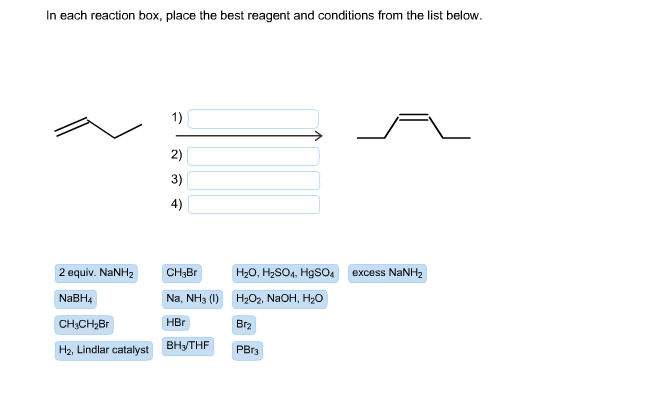In each reaction box, place the best reagent and conditions from the list below. In each reaction box, place the best reagent and conditions from the list below. 2 equiv. NaNHz excess NaNH2 CH3Br H20, H2SO4, HgSO4 Na, NH3 (1) H2O2, NaOH, H2O NaBH4 HBr Br2 CH3CH2Br H2. Lindlar catalyst BH3/THF PBr3

• ### In each reaction box, place the best reagent and conditions from the list below. n each reaction box...In each reaction box, place the best reagent and conditions from the list below. n each reaction box, place the best reagent and conditions from the list below. The third step is correct, but the others are not. PCC oxidizes an alcohol to a carbonyl group. Which reagents will convert an alkene to a hydroxyl group, and with the necessary regioselectivity? 2) NaBH in EtOH 3) PCC in CH2Cl2 PhCO3H in CH2012 CH30Na in CH3OH B2H6 in THF in CH2Cl2...

• ### In each reaction box, place the best reagent and conditions from the list below. In each...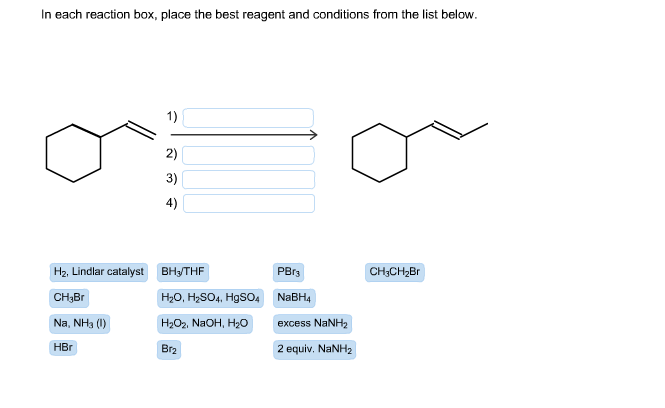In each reaction box, place the best reagent and conditions from the list below. In each reaction box, place the best reagent and conditions from the list below 1) 2) 3) 4) PBrs H2, Lindlar catalyst Вн-THF CH5CH2Br NaBH4 CH3Br H2O, H2SO4, HgSO4 Na, NHa () НаОг, NaOH, Hzо excess NaNH2 HBr Br2 2 equiv. NaNH2

• ### In each reaction box, place the best reagent and conditions from the list below. In each...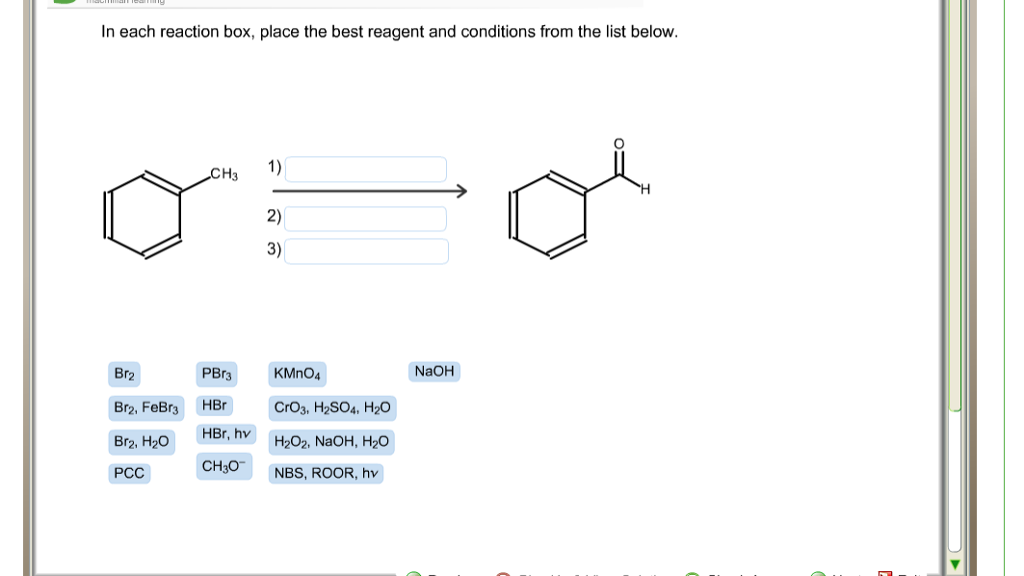In each reaction box, place the best reagent and conditions from the list below. In each reaction box, place the best reagent and conditions from the list below. 1) H3 2) 3) Br2 PBr3 KMnO4 NaOH Br2, FeBr3HBr CrO3. H2S04, H2O HBr, h H202, NaOH, H20 CH30 NBS, ROOR, hv PCC

Free Homework App